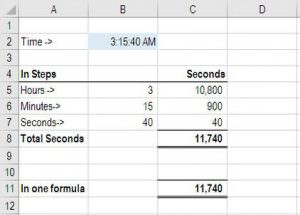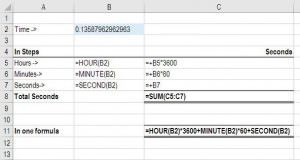Convert Time to Seconds in Excel

This article outlines how to convert time to seconds in Excel.

Excel convert time to seconds

It may be useful to convert the time in Excel from the standard hours and minutes format to seconds only.  In order to do this, there are two main methods.  The best method is to sum the formulas HOUR, MINUTE, and SECOND to get the total seconds. The following methods determine how many seconds have elapsed from midnight (12 AM).  Both methods have Excel convert time to seconds.

Example of how to convert time to seconds in Excel

Here is a step-by-step breakdown of how to have Excel convert time to seconds:

(See screenshots below)

If we take the example time as being 1:40:30 AM and want to figure out how many seconds that is, we can use the following approach.

Step 1 Make sure the time is formatted properly – go to Format Cells (press Ctrl + 1) and make sure the number is set to Time.

Step 2 Use the =HOUR(B2) formula to get the number of hours from the time and multiply by 3600 (there are 3600 seconds in every hour)

Step 3 Use the =MINUTE(B2) formula to get the number of minutes from the time and multiply by 60 (60 seconds in every minute)

Step 4 Use the =SECOND(B2) formula to get the number of seconds from the time

Step 5 Add up the three pieces to get the total seconds

Step 6 Make sure it’s formatted properly as a Decimal, and not as Time (press Ctrl +1 and select Decimal)

Congratulations, you have now had Excel convert time!More Excel learning

This has been CFI’s guide on how to have Excel convert time, using two different methods.  To keep learning and developing your skills, we highly recommend our Free Excel Crash Course which will teach you all the foundational skills you need.  We highly recommend these free guides below:

• Excel for dummies
• Index and Match
• Excel shortcuts
• Excel formulas list
• What is financial modeling

Free Excel Tutorial

To master the art of Excel, check out CFI’s FREE Excel Crash Course, which teaches you how to become an Excel power user.  Learn the most important formulas, functions, and shortcuts to become confident in your financial analysis.

Launch CFI’s Free Excel Course now to take your career to the next level and move up the ladder!